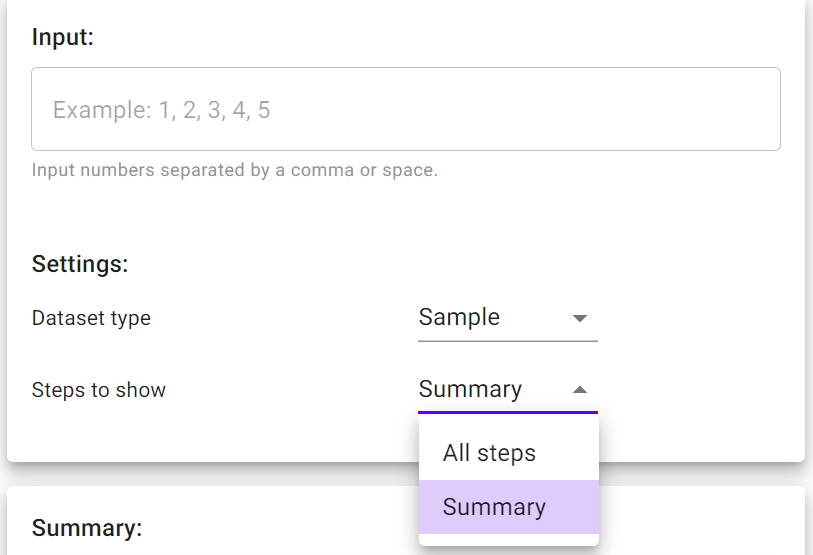# Standard Deviation Calculator

Feb 11, 2023 1:40 PM

Are you looking for a tool to calculate the standard deviation of the dataset you collected in your research or for any other purposes? Check out Calculatorful's standard deviation calculator.

## How to use Calculatorful's standard deviation calculator?

Calculatorful's standard deviation calculator is developed to optimize your data entry process as much as possible. To calculate the standard deviation of your dataset, you need to follow some simple steps:

1. Select the type of your dataset. Is it a population or is it a sample?
2. Select what you want to show in the result, all steps or only a summary
3. Enter your dataset using commas or spaces to separate your data points,
4. And you can see the result right under it.Notice that you can change the order of Step 1, Step 2, and Step 3.

You may already know why you need to calculate the standard deviation of your dataset, but why don't we just discuss a bit more about this widely statistical measure?

## What's standard deviation?

Standard deviation (SD) calculated as the square root of the variance is a measure in statistics describing the dispersion of the data from its mean. The higher the standard deviation, the more widely the dataset spread. A low standard deviation value shows that the data points tend to gather close to their mean. Whether a high standard deviation or a low standard is better? The answer depends on what we expect.

For example, a researcher uses a Likert scale to assess the customers’ satisfaction with a product from 1 to 5 with 1 as the least satisfied and 5 as the highest satisfied. If the mean of the dataset is 4 with a standard deviation of 1, then most customers rate 3,4,5. However, with the same mean of 4, the standard deviation is 2, which means that the customers’ satisfaction is more dispersed and it’s likely that there are more customers who are dissatisfied with the product. In this case, a high standard deviation isn’t desired.

In another example, the mean of the student’s scores on a test is 5 with 10 as the highest grade, and the value of standard deviation is 1, which means that most students score 4,5,6. With a higher standard deviation of 3, the score range is wider, which shows that the test better measures the students’ performance, and better classifies the students into different groups. In this case, the teacher expects a mean of 5 and a high standard deviation.

## How to find standard deviation?

### Population standard deviation

We calculate population standard deviation when all the population is measured. For example, a school may want to know the standard deviation of the math placement test scores of all its students, then the population standard deviation needs to be calculated.

Population standard deviation ($\sigma$) is calculated as the squared root of the variance (${\sigma }^{2}$):

$\sigma =\sqrt{{\sigma }^{2}}=\sqrt{\frac{1}{N}\sum _{i=1}^{N}{\left({X}_{i}-\mu \right)}^{2}}$

Where

xi is an individual value
μ is the mean/expected value
N is the total number of values

For example, the population N is a class of 8 students with test scores of 2, 2, 4, 4, 4, 5, 5, 7, and 9 each.
First of all, we need to calculate the mean/expected value.

$\mu =\frac{2+4+4+4+5+5+7+9}{8}=\frac{40}{8}=5$

To simplify the variance calculation, we calculate the differences between each score and the mean, then square the results
${\left(2-5\right)}^{2}={\left(-3\right)}^{2}=9\phantom{\rule{0ex}{0ex}}{\left(4-5\right)}^{2}={\left(-1\right)}^{2}=1\phantom{\rule{0ex}{0ex}}{\left(4-5\right)}^{2}={\left(-1\right)}^{2}=1\phantom{\rule{0ex}{0ex}}{\left(4-5\right)}^{2}={\left(-1\right)}^{2}=1$             ${\left(5-5\right)}^{2}={0}^{2}=0\phantom{\rule{0ex}{0ex}}{\left(5-5\right)}^{2}={0}^{2}=0\phantom{\rule{0ex}{0ex}}{\left(7-5\right)}^{2}={2}^{2}=4\phantom{\rule{0ex}{0ex}}{\left(9-5\right)}^{2}={4}^{2}=16$

According to the above formula, the variance is then calculated as follows:

${\sigma }^{2}=\frac{9+1+1+1+0+0+4+16}{8}=\frac{32}{8}=4$

Now we take the squared root of the variance to get the value of the standard deviation:

$\sigma =\sqrt{4}=2$

However, in most cases, we can't measure the whole population, say all customers of a product, so we have to sample the population. That's why we have the sample standard deviation.

### Sample standard deviation

Sampling is a scientific approach that is often adopted when it's impossible to get the population. A sample then represents the whole population, and the sample standard deviation is calculated to infer the variability of the population. When using a sample date, we need to correct the formula to avoid underestimating the diversity of the population by replacing N with N -1.

$s=\sqrt{{s}^{2}}=\sqrt{\frac{1}{n-1}\sum _{i=1}^{N}{\left({x}_{i}-\overline{x}\right)}^{2}}$

Where

xi is one sample value
is the sample mean
N is the sample size

## Conclusion

This Calculatorful's standard deviation calculator provides you with a simple and easy-to-approach tool to calculate standard deviation. You also can read the article to know how to calculate SD by hand.Calculator

Input:

Input numbers separated by a comma.

Settings:

Dataset type

Steps to show

Summary:

 Observations (N): 0 0 0Calculatorful

This website is responsive, user friendly and provides calculators that suit every calculational needs in every subject and domain such as maths, finance, physics, sports, food, health, and many others.